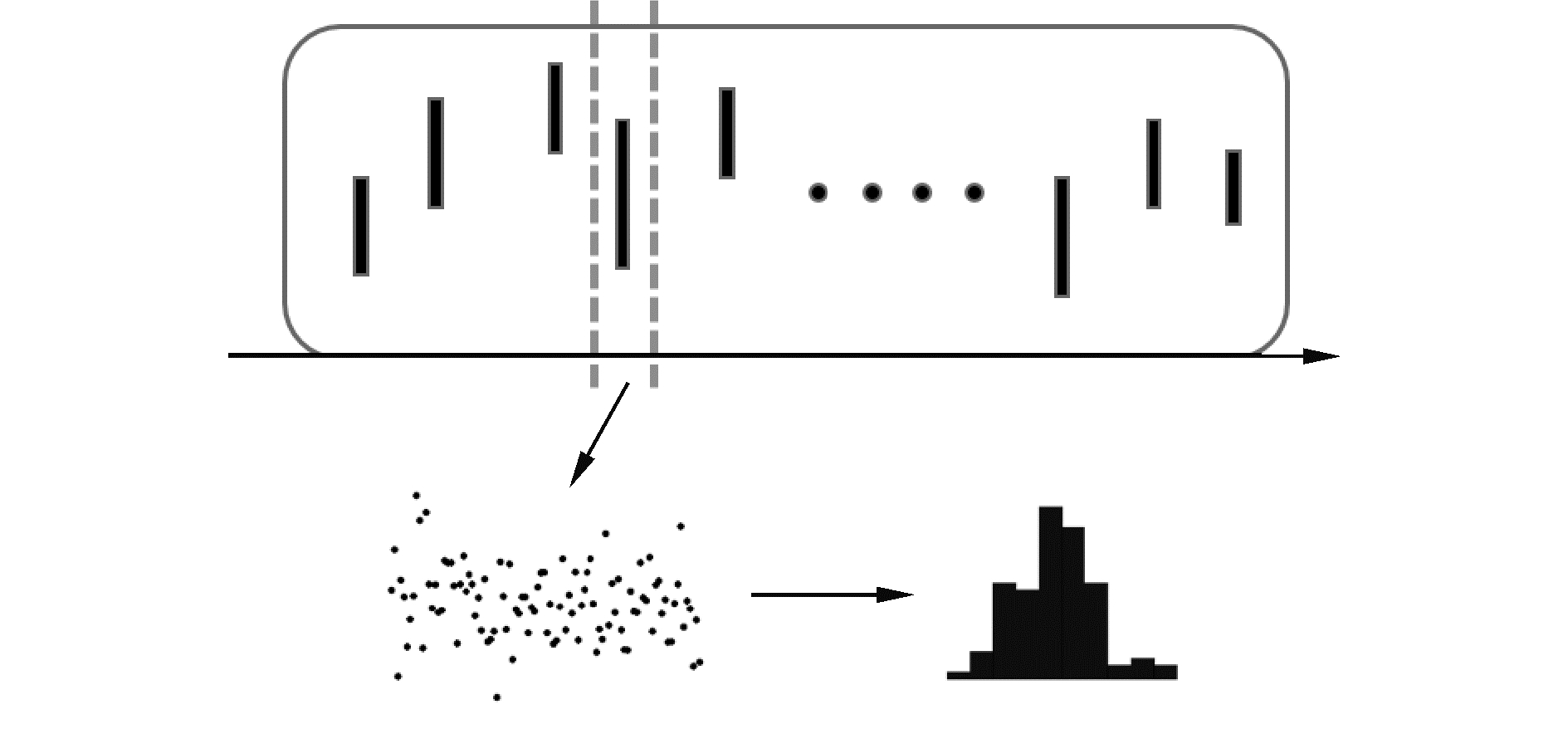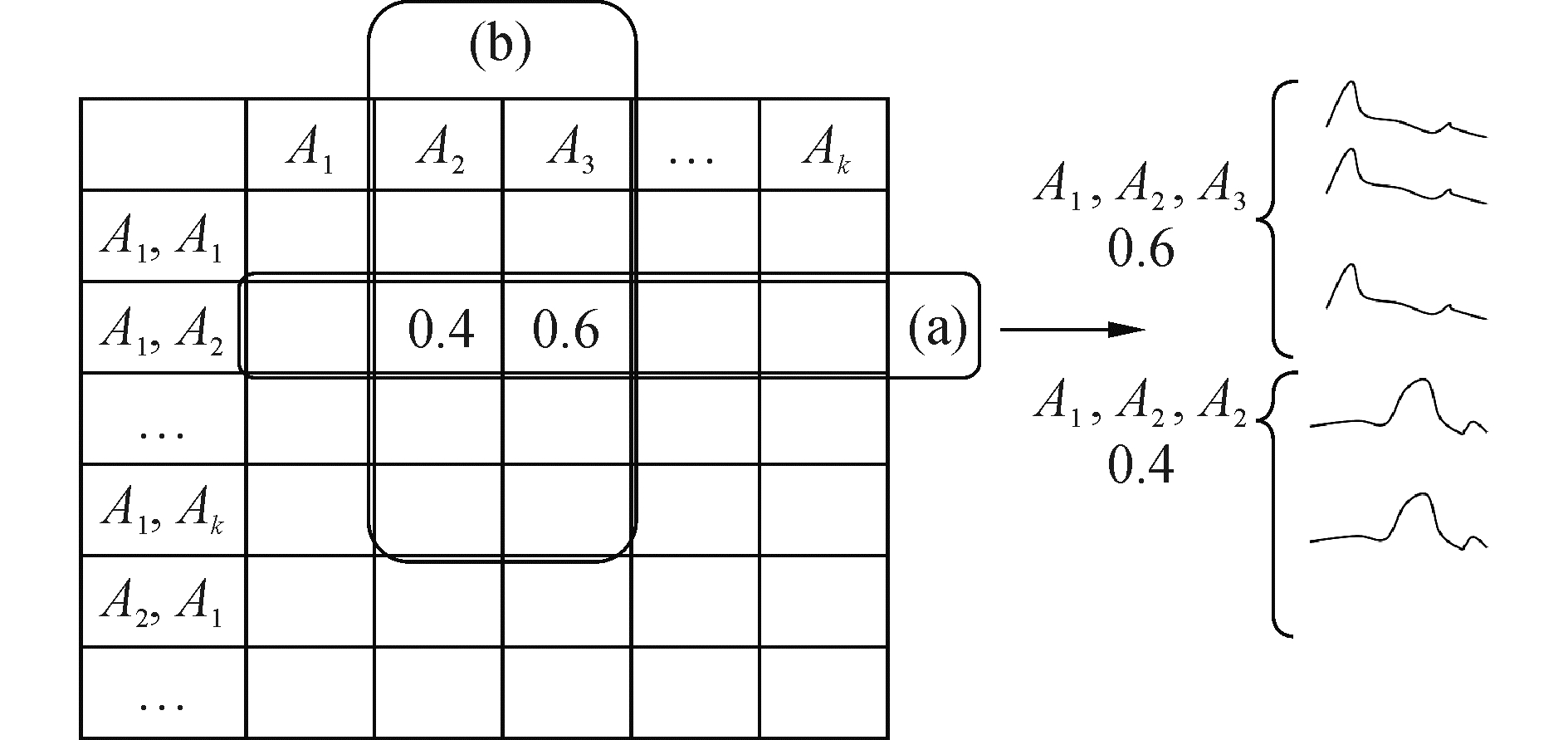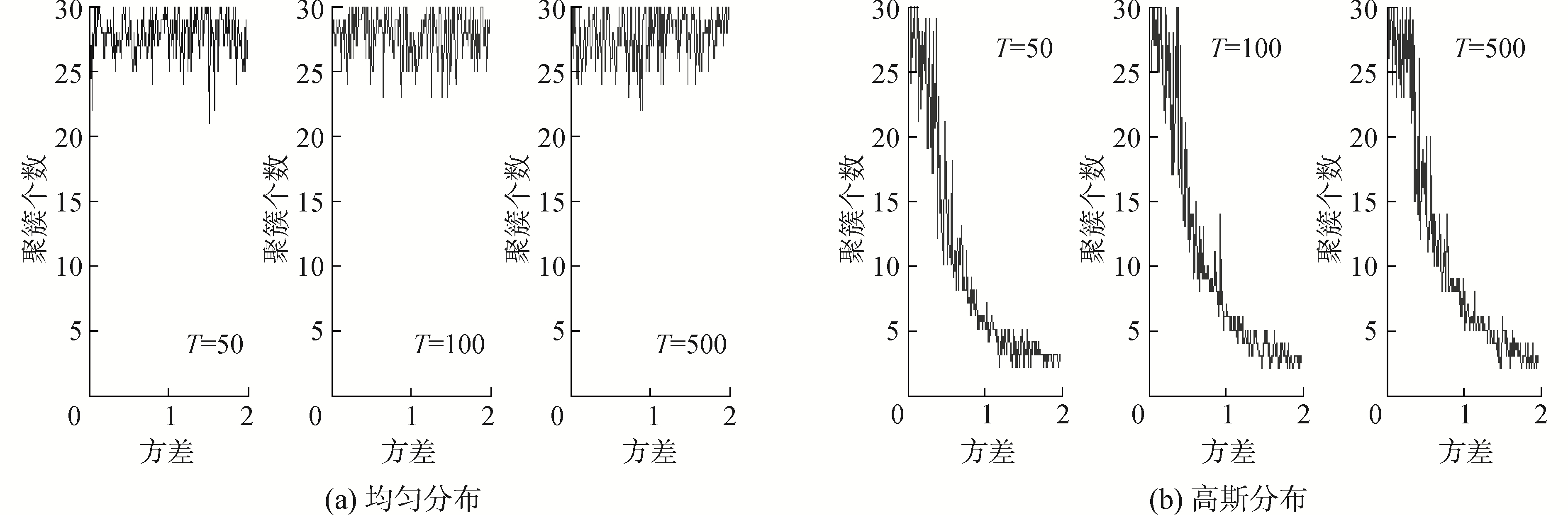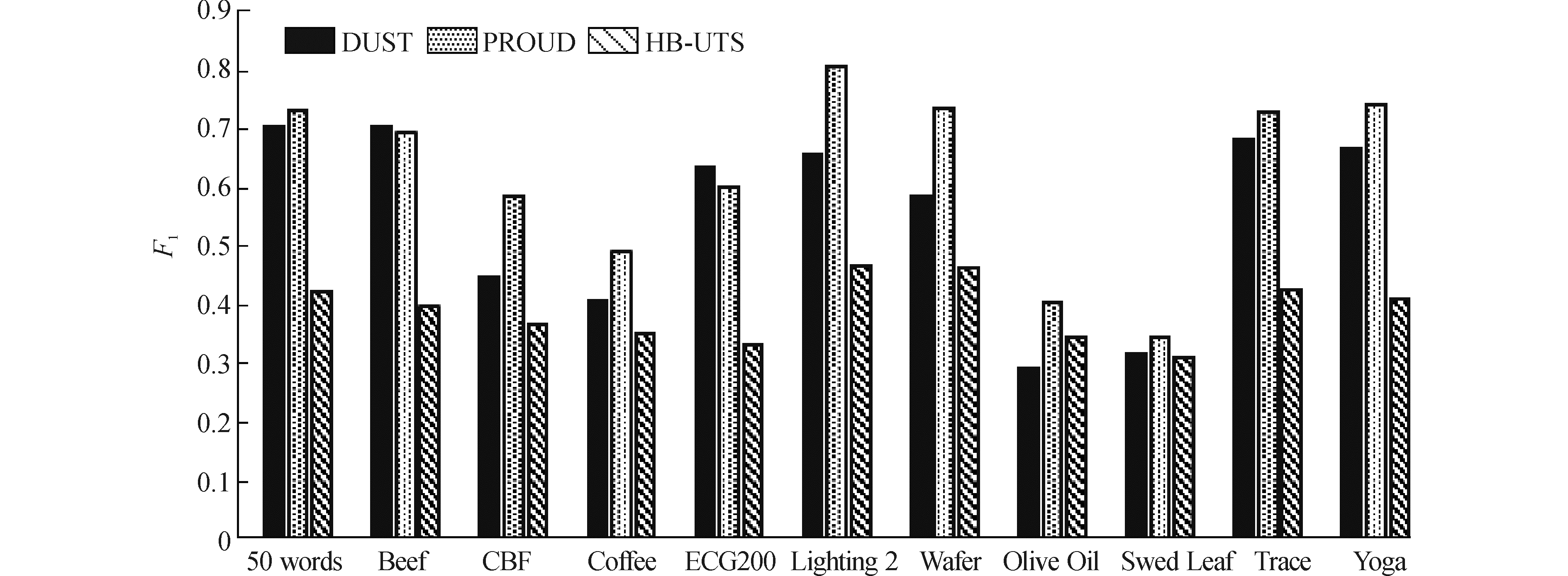﻿ 基于频繁密度分布模式的不确定数据流查询方法
«上一篇文章快速检索 高级检索

 哈尔滨工程大学学报2018, Vol. 39Issue (6): 1052-1058  DOI: 10.11990/jheu.2017070940

### 引用本文CHI Ronghua, HUANG Shaobin, LYU Tianyang. Query processing on uncertain data stream based on frequency density distribution pattern[J]. Journal of Harbin Engineering University, 2018, 39(6), 1052-1058. DOI: 10.11990/jheu.201707094.### 文章历史

1. 哈尔滨工程大学 计算机科学与技术学院, 黑龙江 哈尔滨 150001;
2. 审计署计算机技术中心, 北京 100073

Query processing on uncertain data stream based on frequency density distribution pattern
CHI Ronghua1, HUANG Shaobin1, LYU Tianyang2
1. College of Computer Science and Technology, Harbin Engineer University, Harbin 150001, China;
2. Audit Research Institute of Chinese National Audit Office, Beijing 100073, China
Abstract: To solve the defect of inaccurate modeling for uncertain objects in processing similarity query of uncertain data streams, HB-UTS method was proposed. Non-parametric estimation is used to model the uncertain objects to obtain the density function. The frequency pattern of density function is mined by spectral clustering method and the mined object pattern is abstracted as an indefinite semantic data stream sequence. In the similarity query phase, an index structure of the uncertain data stream is constructed by the state transition matrix model of high-order Markov. It also records the storage probability of the sequence elements while recording the storage address of the uncertain data stream to improve the step-by-step input query efficiency of data stream. To analyze the effect of this method in practical problems, a method combining reality and simulation was adopted. By the experiments on the randomized real dataset and comparing with other similarity query methods, it was verified that HB-UTS is very effective in processing large-scale uncertain data stream.
Key words: uncertainty    data stream    similarity query    non-parametric estimation    data mining    Markov

1 不确定数据流相似性查询方法

1.1 不确定数据流的密度分布模型Download: 图 1 不确定数据流的非参数建模方法示意图 Fig. 1 Non-parametric estimation modeling for uncertain data stream

 ${{\hat f}_h}\left( x \right) = \left\{ \begin{array}{l} \frac{{{v_i}}}{{nh}}, \;\;\;\;I\left( {x \in B} \right)\\ 0, \;\;\;\;\;\;\;{\rm{others}} \end{array} \right.$ (1)

1) 给定一个不确定对象的样本ui, jl，大小为l，对其排序并设x1=min(ui, jl)以及xn=max(ui, jl)分别为最大和最小样本，确定最小下届a0x1

2) 估计组距h，可得组分的界点a0, a1, …, ak，其中ai+1-ai=hi=0, 1, …, k-1，xnakxn+h;

3) 计算落在每组区间Ai=(ai, ai+1]，i=0, 1, …, k-1中的样本频数:v1, v2, …, vk

4) 以h为带宽，v1, v2, …, vk为高作直方图；

5) 由直方图估计样本对应总体的密度分布可得${{\hat f}_h}\left( x \right)$

 $R\left( {\hat u, u} \right) = \smallint {\left( {\hat u\left( x \right) - u\left( x \right)} \right)^2}{\rm{d}}x$ (2)

 $R\left( {\hat u, u} \right) = {\rm{E}}\smallint {\left( {\hat u\left( x \right) - u\left( x \right)} \right)^2}{\rm{d}}x$ (3)
 $\begin{array}{l} R, \left( {\hat u, u} \right) = \smallint {\left( {{\rm{E}}\hat u\left( x \right) - u\left( x \right)} \right)^2}{\rm{d}}x + \\ \;\;\;\;\;{\rm{E}}\smallint {\left( {\hat u\left( x \right) - u\left( x \right)} \right)^2}{\rm{d}}x \end{array}$ (4)
 $R\left( {\hat u, u} \right) = \smallint {\rm{Bia}}{{\rm{s}}^2}\left( x \right){\rm{d}}x + \smallint V\left( x \right){\rm{d}}x$ (5)

 $\begin{array}{l} \smallint {\rm{Bia}}{{\rm{s}}^2}\left( x \right){\rm{d}}x = \smallint {\left( {u'\left( x \right)} \right)^2}\left( {\frac{h}{2} - {x^2}} \right){\rm{d}}x\\ \;\;\;\smallint {\rm{Bia}}{{\rm{s}}^2}\left( x \right){\rm{d}}x \approx \frac{{{h^2}}}{{12}}\smallint u'{\left( x \right)^2}{\rm{d}}x \end{array}$ (6)

 $V\left( x \right) = \frac{{{u_j}}}{{n{h^2}}} \approx u\left( x \right)/nh,$ (7)

 $R\left( {\hat u, u} \right) \approx \frac{{{h^2}}}{{12}}\smallint {\left( {u'\left( x \right)} \right)^2}{\rm{d}}x + \frac{1}{{nh}}$ (8)

 ${h^*} = \frac{1}{{{n^{\frac{1}{3}}}}}{\left( {\frac{6}{{\smallint u'{{\left( x \right)}^2}{\rm{d}}x}}} \right)^{1/3}}$ (9)

1.2 不确定对象的频繁密度分布模式挖掘

1) 计算S的相似矩阵Q，根据高斯相似性有qij=exp(-‖${{\mathit{\boldsymbol{\hat u}}}_i} - {{\mathit{\boldsymbol{\hat u}}}_j}$2/2δ2), ij, qij=0；

2) 构造拉普拉斯矩阵L=D-Q，其中D为对角矩阵, 且${d_{ii}} = \sum\limits_{k = 1}^n {{q_{ik}}}$

3) 计算拉普拉斯矩阵L的特征值，将其升序排列0＜λ1λ2≤…≤λn

4) 计算特征值序列的差分，当差分的方差明显变化时得k个特征值及其对应的特征向量；

5) 利用前k个特征向量构成矩阵，再通过k-means聚类方法聚类；

6) 最后，得到k个聚簇的聚类结果。

1.3 基于语义关系的不确定数据流查询方法

1.3.1 不确定数据流的模糊语义关系表示

1.3.2 不确定数据流查询方法

 $\begin{array}{l} \begin{array}{*{20}{c}} {}&{{A_1}}&{{A_2}}& \cdots &{{A_k}} \end{array}\\ \begin{array}{*{20}{c}} {{A_1}, {A_1}}\\ {{A_1}, {A_2}}\\ \vdots \\ {{A_k}, {A_k}} \end{array}\left[ {\begin{array}{*{20}{c}} {{a_{1, 1, 1}}}&{{a_{1, 1, 2}}}& \cdots &{{a_{1, 1, k}}}\\ {{a_{1, 2, 1}}}&{{a_{1, 2, 2}}}& \cdots&\vdots \\ \vdots&\vdots&\ddots &{{a_{\left( {k - 1} \right), \left( {k - 1} \right), k}}}\\ {{a_{k, k, 1}}}& \cdots &{{a_{k, k, (k - 1)}}}&{{a_{k, k, k}}} \end{array}} \right] \end{array}$

a1, 1, 1是语义关系的一种统计，则可进行归一化处理使${a_{ijh}} = {a_{ijh}}/\sum\limits_{h = 1}^k {{a_{ijh}}}$Download: 图 2 二阶Markov状态转移矩阵 Fig. 2 Second order Markov transition matrix

2 实验分析

2.1 实验思路表 1 数据流数据集 Tab.1 Data stream datasets

2.2 实验结果分析Download: 图 3 不同不确定性条件下的聚簇个数变化 Fig. 3 Cluster numbers with different uncertaintyDownload: 图 4 高斯分布随机化后的F1值对比 Fig. 4 F1 comparison of Gaussian distribution randomizationDownload: 图 5 高斯分布与均匀分布随机化后的F1值对比 Fig. 5 F1 comparison of mixture of Gaussian and uniform distribution randomization
3 结论

1) 本方法可对属性级以及存在级的不确定数据流建模，有效保存其中的不确定性信息；

2) 基于频繁模式挖掘以及模糊语义的方法，可识别数据流的序列模式，并通过Markov索引进行快速匹配；

3) 通过随机化仿真实验，可知HB-UTS对不同规模的不确定数据流都具有稳定和较小的模型复杂性，在处理大规模数据时也可以确保算法的时效性；

4) 当样本的不确定性体现为密度分布模式相同或者不确定对象间的密度分布模式具有规律时，HB-UTS相对于其他的相似性查询方法具有较好的查询准确性，而当这种模式为完全随机时，查询效果则较差。

  RE C, DALVI N, SUCIU D. Efficient top-k query evaluation on probabilistic data[C]//Proceedings of the 23rd International Conference on Data Engineering. Istanbul, Turkey, 2007: 886-895. (0)  JEFFERY S R, GAROFALAKIS M, FRANKLIN M J. Adaptive cleaning for RFID data streams[C]//Proceedings of the 32nd International Conference on Very Large Data Bases. Seoul, Korea, 2006: 163-174. http://dl.acm.org/citation.cfm?id=1164143 (0)  TRAN T, SUTTON C, COCCI R, et al. Probabilistic inference over RFID streams in mobile environments[C]//Proceedings of the 25th IEEE International Conference on Data Engineering. Shanghai, China, 2009: 1096-1107. http://dl.acm.org/citation.cfm?id=1546683.1547385 (0)  CHEN Lei, ÖZSU M T, ORIA V. Robust and fast similarity search for moving object trajectories[C]//Proceedings of 2005 ACM SIGMOD International Conference on Management of Data. Baltimore, Maryland, 2005: 491-502. http://dl.acm.org/citation.cfm?id=1066213 (0)  LJOSA V, SINGH A K. APLA: indexing arbitrary probability distributions[C]//Proceedings of the 23rd IEEE International Conference on Data Engineering. Istanbul, Turkey, 2007: 946-955. http://doi.ieeecomputersociety.org/10.1109/ICDE.2007.367940 (0)  SARMA A D, BENJELLOUN O, Halevy A, et al. Working models for uncertain data[C]//Proceedings of the 22nd International Conference on Data Engineering. Atlanta, GA, USA, 2006. http://dl.acm.org/citation.cfm?id=1129867 (0)  WIDOM J. Trio: a system for integrated management of data, accuracy, and lineage[C]//Proceedings of the Biennial Conference on Innovative Data Systems Research., 2005: 262-276. http://www.researchgate.net/publication/220988230_Trio_A_System_for_Integrated_Management_of_Data_Accuracy_and_Lineage (0)  FUXMAN A, FAZLI E, MILLER R. ConQuer: efficient management of inconsistent databases[C]//Proceedings of 2005 ACM SIGMOD International Conference on Management of Data. Baltimore, Maryland, 2005: 155-166. http://dl.acm.org/citation.cfm?id=1066176 (0)  DALVI N, SUCIU D. Efficient query evaluation on probabilistic databases[J]. The VLDB journal, 2007, 16(4): 523-544. DOI:10.1007/s00778-006-0004-3 (0)  SOLIMAN M A, ILYAS I F, CHANG K C C. URank: formulation and efficient evaluation of top-k queries in uncertain databases[C]//Proceedings of 2007 ACM SIGMOD International Conference on Management of Data. Beijing, China, 2007: 1082-1084. http://dl.acm.org/citation.cfm?id=1247613 (0)  赵越, 王意洁, 王媛, 等. 一种高效的不确定数据流并行Skyline查询处理方法[J]. 计算机研究与发展, 2013, 50(S1): 132-139. ZHAO Yue, WANG Yijie, WANG Yuan, et al. An efficient method of parallel skyline query processing over uncertain data stream[J]. Journal of computer research and development, 2013, 50(S1): 132-139. (0)  ZHOU Xu, LI Kenli, ZHOU Yantao, et al. Adaptive processing for distributed skyline queries over uncertain data[J]. IEEE transactions on knowledge and data engineering, 2016, 28(2): 371-384. DOI:10.1109/TKDE.2015.2475764 (0)  CICERI E, FRATERNALI P, MARTINENGHI D, et al. Crowdsourcing for top-K query processing over uncertain data[J]. IEEE transactions on knowledge and data engineering, 2016, 28(1): 41-53. DOI:10.1109/TKDE.2015.2462357 (0)  RAMÍREZ-GALLEGO S, KRAWCZYK B, GARCÍA S, et al. A survey on data preprocessing for data stream mining:current status and future directions[J]. Neurocomputing, 2017, 239: 39-57. DOI:10.1016/j.neucom.2017.01.078 (0)  张晨, 金澈清, 周傲英. 一种不确定数据流聚类算法[J]. 2010, 21(9): 2173-2182. ZHANG Chen, JIN Cheqing, ZHOU Aoying. Clustering algorithm over uncertain data streams[J]. Journal of software, 2010, 21(9): 2173-2182. http://kns.cnki.net/KCMS/detail/detail.aspx?filename=rjxb201009008&dbname=CJFD&dbcode=CJFQ (0)  DALLACHIESA M, NUSHI B, MIRYLENKA K, et al. Uncertain time-series similarity:return to the basics[J]. Proceedings of the VLDB endowment, 2012, 5(11): 1662-1673. DOI:10.14778/2350229 (0)